# Transition-operator semi-group

(diff) ← Older revision | Latest revision (diff) | Newer revision → (diff)

The semi-group of operators generated by the transition function of a Markov process. From the transition function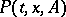of a homogeneous Markov processin a state space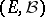one can construct certain semi-groups of linear operators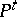acting in some Banach space. Very often,is the space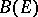of bounded real-valued measurable functionsinwith the uniform norm (or for a Feller process, the space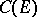of continuous functions with the same norm) or else the spaceof finite countably-additive functionsonwith the complete variation as norm. In the first two cases one putsand in the third(hereandbelong to the corresponding spaces,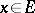,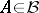). In all these cases the semi-group property holds: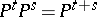,, and any of the three semi-groups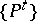is called a transition-operator semi-group.

In what follows, only the first case is considered. The usual definition of the infinitesimal generatorof the semi-group(this is also the infinitesimal generator of the process) is as follows:for all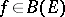for which this limit exists as a limit in. It is assumed thatforis a measurable function of the pair of variables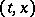, and one introduces the resolvent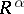of the process,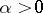, by: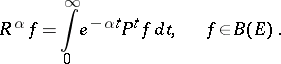(*)

Ifas, then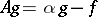, where. Under certain assumptions the integral (*) exists also for, and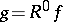satisfies the "Poisson equation"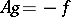(for this reason, in particular,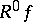is called the potential of).

Knowledge of the infinitesimal generator enables one to derive important characteristics of the initial process; the classification of Markov processes amounts to the description of their corresponding infinitesimal generators , . Also, using the infinitesimal generator one can find the mean values of various functionals. For example, under certain assumptions the function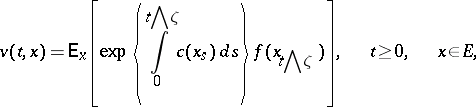is a unique solution to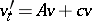,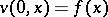, which is a not-too-rapidly-increasing function of. Here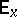is the mathematical expectation corresponding to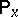, while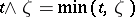.

The operatoris related to the characteristic operator. Letbe a Markov process that is right continuous in a topological space. For a Borel functionone putsif the limit exists for all, whereruns through a system of neighbourhoods of the pointcontracting towardsand whereis the moment of first exit offrom(if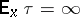, the fraction in the limit is set equal to zero). In many cases the calculation of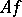amounts to calculating.

How to Cite This Entry:
Transition-operator semi-group. Encyclopedia of Mathematics. URL: http://encyclopediaofmath.org/index.php?title=Transition-operator_semi-group&oldid=17099
This article was adapted from an original article by M.G. Shur (originator), which appeared in Encyclopedia of Mathematics - ISBN 1402006098. See original article